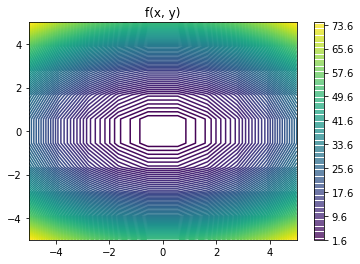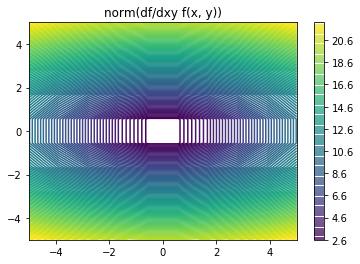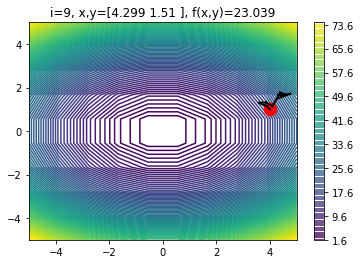In this exercise you will:

1. Write you function $f(x, y)$
2. Use gradient descent with and without momentum
3. Using your implementation, optimize $f(x, y)$ w.r.t. the vector $[x, y]^T$.
4. Redo step 1 and 3 to optimize a different function
5. Write a short description of the sensitivity of the two algorithms to their hyperparemeter values

After that, you're done. If you want more, you can try one of the following stretch goals:

1. In practice our gradient updates are often noisy (see the stochastic GD algorithm). Add a small amount of noise to the gradient update. If g=autograd.grad(f)(xy), this would look like g+=.2*np.random.randn(*g.shape) within your get_gd_delta and get_gd_delta_mom functions. How does performance of GD and GD-with-momentum respond when gradients are noisy?
2. Read the documentation of scipy.optimize.check_grad. Use this method to write a unit test of autograd.grad(f) and test it out for some non-trivial functions.
3. Read section 2 of the Adam paper. Implement Adam from scratch by following algorithm 1 or translate an existsing online implementation or tutorial into autograd.
In :
import autograd.numpy as np
import matplotlib.pyplot as plt
%matplotlib inline
np.set_printoptions(precision=3)
import time

# helper function for plotting isocontours
def plot_isocontours(x_points, y_points, g):
"""
first makes a 2d grid from the 1d grid
then plots isocontours using the function g
"""
X,Y = np.meshgrid(x_points, y_points)  # build 2d grid
Z = np.zeros_like(X)
for i in range(len(X)):
for j in range(len(X.T)):
Z[i, j] = g(np.array((X[i, j], Y[i, j])))  # compute function values
fig, ax = plt.subplots()
im = ax.contour(X, Y, Z, 100)
plt.colorbar(im, ax=ax)
return fig, ax


# 1a. Write your own function f(x, y) using the template below.¶

x and y are represented by the 2d vector xy

In :
# write forward function
def f(xy):
x, y = xy, xy

# write your own function by changing the expression below
output = x**2 + 2.*y**2  # an easy one
# output = x**2 + 2.*y**2 + 2.*x*y  # a weird one
# output = np.log(x**2 + 0.25*y**2) + 0.01*x**2*y**2  # ???
# write your own function by changing the expression above

# remember that only convex functions have well-definied and unique global minima
return output

# differentiate f(x) w.r.t. x


# 1b. plot isocontours of $f(x, y)$ and $\nabla_{x, y}||f(x, y)||_2$¶

In :
x_points = np.linspace(-5, 5, 10)  # optionally change the plot limits here
y_points = np.linspace(-5, 5, 10)

df_norm = lambda xy: np.sqrt(np.sum(np.square(df(xy))))

fig, ax = plot_isocontours(x_points, y_points, f)
ax.set_title('f(x, y)')
fig, ax = plot_isocontours(x_points, y_points, df_norm)
ax.set_title('norm(df/dxy f(x, y))')
plt.show()# 2. Implement the updates for gradient descent with and without momentum using the code template provided¶

In :
def get_gd_delta(xy, f, learning_rate):
"""
xy <-- xy + delta
delta depends on the current position xy, the function f, and the learning rate
"""
# write your update code below
delta = -learning_rate*np.random.randn(*xy.shape)  # random upates; replace me
# write your update code above
return delta

def get_gd_mom_delta(xy, f, learning_rate, prev_delta, alpha):
"""
xy <-- xy + delta
delta depends on the current position xy, the function f, the learning rate, the previous delta, and alpha
"""
# write your update code below
delta = -learning_rate*np.random.randn(*xy.shape)  # random upates; replace me
# write your update code above
return delta


# 2 use your implementation to optimize f¶

In :
fig, ax = plot_isocontours(x_points, y_points, f)  # plot function isocontours

# hyperparameters
#INITIAL_VAL = np.random.randn(2)
INITIAL_VAL = np.array([4., 1.])
USE_MOMENTUM = True
LEARNING_RATE = 0.3
ALPHA = 0.8
N_ITER = 10  # see if you can reach the function minimum in as few iterations as possible

# initialize
xy = np.copy(INITIAL_VAL)
delta = np.zeros(2)
ax.plot(*xy, color='r', marker='.', ms=25)  # plot initial values

from IPython import display

for i in range(N_ITER):
#    input("Press Enter to continue...")  # optional; Enter key triggers next update
#    time.sleep(0.5)  # optional; slow down animation if the flickering is distracting

xy_old = np.copy(xy)
g = df(xy)  # compute standard gradient
if USE_MOMENTUM:
delta = get_gd_mom_delta(xy, f, LEARNING_RATE, delta, ALPHA)
else:
delta = get_gd_delta(xy, f, LEARNING_RATE)
xy += delta  # update position

# plot
ax.plot([xy_old, xy], [xy_old, xy],'-k',lw=2)  # plot a line connecting old and new param values
fig.canvas.draw()
ax.set_title('i={}, x,y={}, f(x,y)={:.3f}'.format(i, xy, f(xy)))
display.display(fig)
display.clear_output(wait=True)

print('final value f(x, y)={:.3f} at (x, y)=({:.3f}, {:.3f})'.format(f(xy), xy, xy))

final value f(x, y)=23.039 at (x, y)=(4.299, 1.510)# 5. Briefly describe what you observed.¶

## How did the optimization change as the values of the hyperparameters (INITIAL_VAL, USE_MOMENTUM, LEARNING_RATE, ALPHA, N_ITER) were adjusted?¶

Double click to write your response here...

In [ ]: